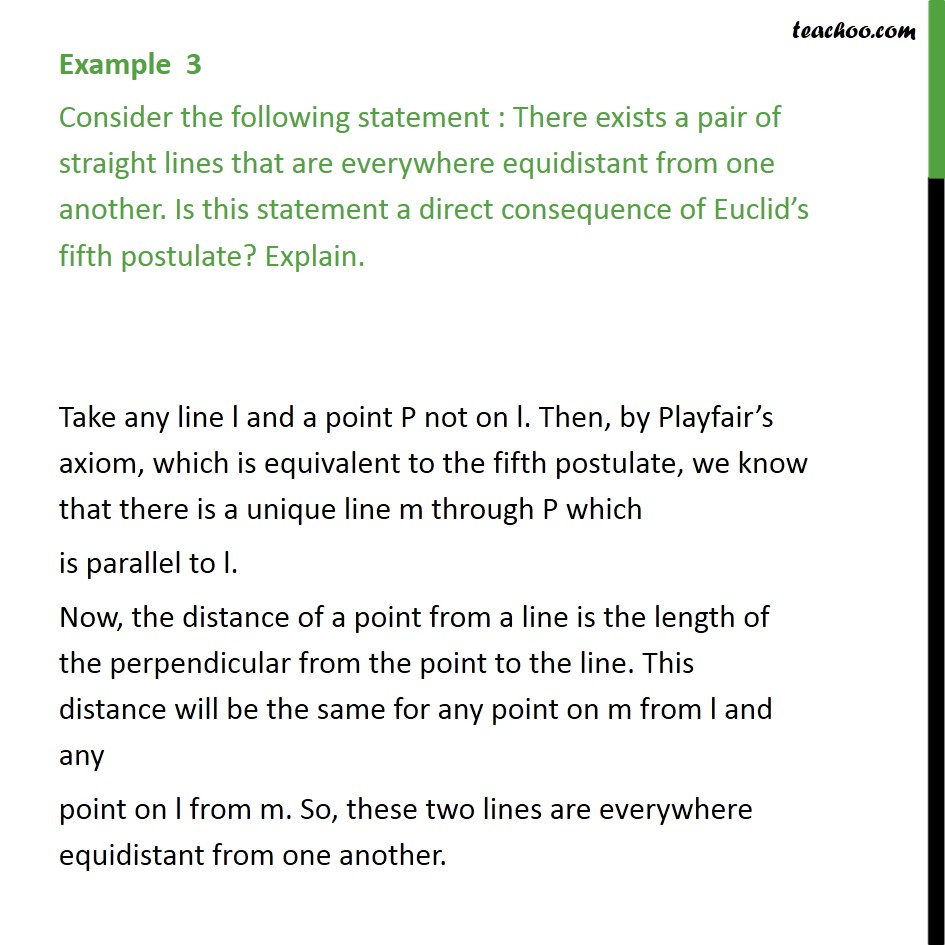Postulates

Chapter 5 Class 9 Introduction to Euclid's Geometry
Concept wiseLearn in your speed, with individual attention - Teachoo Maths 1-on-1 Class

### Transcript

Example 3 Consider the following statement : There exists a pair of straight lines that are everywhere equidistant from one another. Is this statement a direct consequence of Euclid’s fifth postulate? Explain. Take any line l and a point P not on l. Then, by Playfair’s axiom, which is equivalent to the fifth postulate, we know that there is a unique line m through P which is parallel to l. Now, the distance of a point from a line is the length of the perpendicular from the point to the line. This distance will be the same for any point on m from l and any point on l from m. So, these two lines are everywhere equidistant from one another.